# Active Appearance Models¶

#### By Abhijeet Kislay (GitHub ID: kislayabhi)¶

Here we have developed a parameterized face needed by the Active Appearance Model (AAM), where the variability of shape and texture is captured from a representative training set. PCA on shape and texture is applied to produce the face model which describes the trained faces with a photorealistic quality.

## Background¶

AAM is an object model containing statistical information of its shape and texture. It is a powerful way of capturing shape and texture variation from objects. It comes along with an efficient search algorithm that can tell exactly where and how a model is located in a picture frame.

The two models needed to make an AAM are:

• Shape Models
• Texture Models
In :
#following libraries will be used here

#import Opencv library
try:
import cv2
except ImportError:
print "You must have OpenCV installed"

import matplotlib.pyplot as plt
import numpy as np
from modshogun import *
%matplotlib inline

#Use the following function when reading an image through OpenCV and displaying through plt.
def showfig(image, ucmap):
#There is a difference in pixel ordering in OpenCV and Matplotlib.
#OpenCV follows BGR order, while matplotlib follows RGB order.
if len(image.shape)==3 :
b,g,r = cv2.split(image)       # get b,g,r
image = cv2.merge([r,g,b])     # switch it to rgb
imgplot=plt.imshow(image, ucmap)
imgplot.axes.get_xaxis().set_visible(False)
imgplot.axes.get_yaxis().set_visible(False)


### Shape Model¶

As mentioned previously, AAMs require a shape model, and this role is played by Active Shape Models (ASMs). In the following section, we have described the method to build it.

The shape model is generated through the combination of shape variations and for that a training set of annotated images is required. That is we need to have several images marked with points on key positions of a face which outlines the main features.

Few of the training images with the landmark points are shown below. There are 68 landmarks on a face. These are usually marked up by hand and they outline several face features, such as mouth contour, nose, eyes, eyebrows, and face shape since they are easier to track:

In :
plt.rcParams['figure.figsize'] = 17, 7

fig=plt.figure()
for i in xrange(1,4):
image_path="../../../data/AAM/images/%d.jpg"%i
tim_cootes=cv2.imread(image_path)
axes=fig.add_subplot(1,3,i)
data=np.loadtxt('../../../data/AAM/points/%d.pts'%i, usecols=range(2))
x=data[:,0]
y=data[:,1]
axes.plot(x,y,'*')
showfig(tim_cootes, None)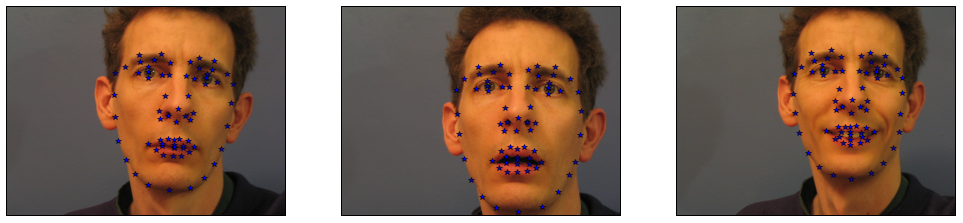The procedures to build an ASM are as follows:

1. Aligning shapes to a common frame using Generalized Procrustes Analysis
2. Modeling the dataset shape variation using PCA

#### Generalized Procrustes Analysis¶

The alignment of two shapes consists on finding the Similarity parameters (scale, rotation and translation) that best match one shape to another by minimizing a given metric. The classical solution of align two shapes is the Procrustes Analysis method. It align shapes with the same number of landmarks with one-to-one point correspondences, which is sufficient for the AAM standard formulation.

The landmark points that we are provided right now are not aligned in a way that helps us to build an ASM. This can be seen in the following figure:

In :
plt.rcParams['figure.figsize'] = 7, 7
figure, axis=plt.subplots(1,1)
plt.xlim(0, 480)
plt.ylim(0, 480)
plt.title("The landmark points for all the face images in the training set")
for i in xrange(1,26):
Y=np.loadtxt('../../../data/AAM/points/%d.pts'%i, usecols=range(2))
axis.plot(640-Y[:,0], 480-Y[:,1],'+', color='blue', markersize=5)
axis.set_xticks([])
axis.set_yticks([])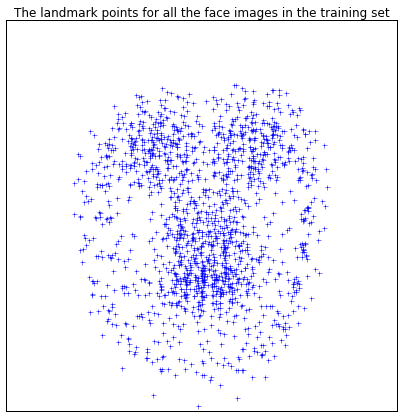A Generalized Procrustes Analysis(GPA) consists of sequentially aligning pairs of shapes with Procrustes using the reference shape (the mean shape) and align others to it.

To facilitate this iterative process, we define a procrustes function below:

In :
def procrustes(X, Y):
"""
Inputs:
------------
X, Y
matrices of target and input coordinates. they must have equal
numbers of  points (rows)

Outputs
------------
Z
the matrix of transformed Y-values
"""

n,m = X.shape
ny,my = Y.shape

muX = X.mean(0)
muY = Y.mean(0)

X0 = X - muX
Y0 = Y - muY

ssX = (X0**2.).sum()
ssY = (Y0**2.).sum()

# centred Frobenius norm
normX = np.sqrt(ssX)
normY = np.sqrt(ssY)

# scale to equal (unit) norm
X0 /= normX
Y0 /= normY

# optimum rotation matrix of Y
A = np.dot(X0.T, Y0)
U,s,Vt = np.linalg.svd(A,full_matrices=False)
V = Vt.T
T = np.dot(V, U.T)

traceTA = s.sum()

# transformed coords
Z = normX*traceTA*np.dot(Y0, T) + muX

return Z


At the beginning any shape can be chosen to be the initial mean. After the alignment a new estimate for the mean is recomputed and again the shapes are aligned to this mean. The procedure is performed repeatedly until the mean shape don't change significantly within iterations. Normally this procedure converges in two iterations.

In :
# ****GPA*****
# choose any shape as the intial mean
X=np.loadtxt('1.pts', usecols=range(2))

# two iterations are enough
for j in xrange(2):
Y_new=[]

#our training set has 25 images numbered 1,2,..,24,25
for i in xrange(1,26):
Y=np.loadtxt('%d.pts'%i, usecols=range(2))
z=procrustes(X, Y)
Y_new.append(z)
#recompute the mean
X=sum(Y_new)/float(len(Y_new))


Lets check the output after applying the GPA over the landmark points that we had:

In :
plt.rcParams['figure.figsize'] = 7, 7
Y_new.shape
figure, axis=plt.subplots(1,1)
plt.title("The GPA processed landmark points")
plt.xlim(0, 480)
plt.ylim(0, 480)
for i in xrange(25):
axis.plot(640-Y_new[i][:,0], 480-Y_new[i][:,1],'+', color='blue', markersize=5)
axis.set_xticks([])
axis.set_yticks([])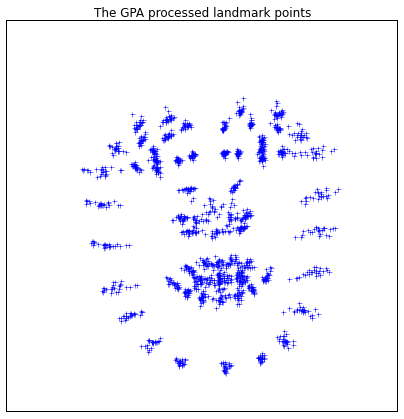After aligning these landmarks using GPA, we will arrange them in the following format where the $k$ aligned landmarks in the two dimensions are given as:

$X = (x_1,y_1,...,x_k,y_k)$

It is important to note that each landmark is consistently labelled across all the training images. So, for instance, if the left part of the mouth is landmark number 3 in the first image, it will need to be number 3 in all the other images.

#### Principal Component Analysis (PCA)¶

The PCA is a statistical technique that allows data dimension reduction. This process searches for directions in the data that has largest variance and subsequently project the data onto it. For more details checkout this notebook on PCA.

Applying PCA on the previously aligned data, the statistical shape variation can be modeled with:

$\mathbf{x}=\mathbf{\bar{x}}+\mathbf{E_s}\mathbf{y_s}$

where new shapes $\mathbf{x}$, are synthesised by deforming the mean shape, $\mathbf{\bar{x}}$, using a weighted linear combination of eigenvectors of the covariance matrix, $\mathbf{E_s}$. $\mathbf{y_s}$ is a vector of shape parameters which represent the weights. $\mathbf{E_s}$ holds $t_s$ most important eigenvectors that explain a user defined variance. It is possible to recover the shape parameters associated with each shape by

$\mathbf{y_s}=\mathbf{E_s}^T(\mathbf{x}-\mathbf{\bar{x}})$

where $\mathbf{y_s}$ vector defines a set of deformable model parameters.

In :
obs_matrix=[]

#arrange the data in the format (x1,y1,...,xk,yk)
for i in xrange(25):
obs_matrix.append(np.hstack(Y_new[i]))

#prepare the observation matrix for PCA
obs_matrix=np.array(obs_matrix).T

#convert it into Shogun RealFeatures format
train_features=RealFeatures(np.array(obs_matrix))

#set the PCA mode to AUTO
preprocessor=PCA(AUTO)

#set the number of eigenvectors to hold onto, here three
preprocessor.set_target_dim(24)

#all set. Run it
preprocessor.init(train_features)
mean=preprocessor.get_mean()

#get the eigenvectors of the covariance marix
Es=preprocessor.get_transformation_matrix()
#get the eigenvalues of the covariance matrix
eigenvalues_s=preprocessor.get_eigenvalues()

#project the data to their principal components
ys=preprocessor.apply_to_feature_matrix(train_features)


The variance of the parameter $y_{si}: i = 1,..., ts$ over the training set is given by $λ_i$. Limiting $y_{si}$ between $±3 \sqrt{λ_i} = ±3σ_i$ is safe that the shape generated is similar to the ones in the training set.

The following images shows the first three shape parameters varied between $[-3\sigma_i, +3\sigma_i]$. The first variation mode, as expected displays more information associated, causing a bigger movement between the landmark position. The lower significatives modes cause a more local variation.

In :
plt.rcParams['figure.figsize'] = 17,5

shape_container=[]

for parameter_no in xrange(3):
std_dev=np.int(eigenvalues_s[parameter_no]**0.5)
figure=plt.figure()
deviation_data=np.array([-3*std_dev, -1.5*std_dev, 0, 1.5*std_dev, 3*std_dev])
title_data=np.array(['-3','-1.5','0','+1.5','+3'])
plot_no=0
for deviation in deviation_data:

plot_no=plot_no+1
new_ys=np.copy(ys)

#vary one of the parameter
new_ys[parameter_no,1]=deviation

# reconstruct the shape landmarks,but with the distorted ys
reconstructed_image=np.hstack(np.dot(Es,new_ys[:,1]))+mean
image=np.resize(reconstructed_image,[68,2])
shape_container.append(image)

axis=figure.add_subplot(1,5,plot_no)
axis.plot(600-image[:,0], 600-image[:,1])
plt.title((title_data[plot_no-1])+' $\sigma %d$'%parameter_no)
axis.set_xticks([])
axis.set_yticks([])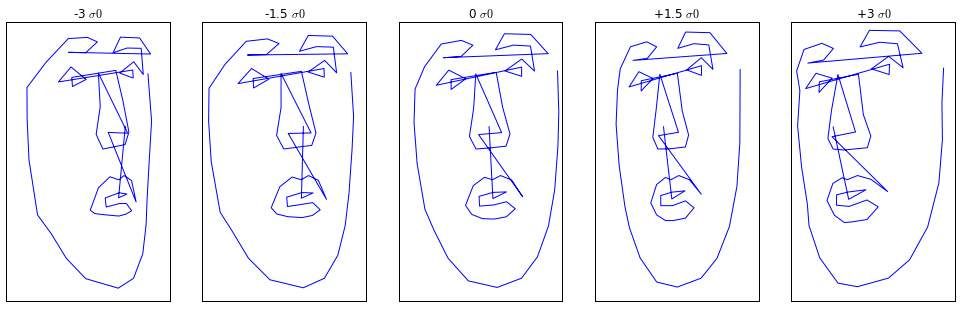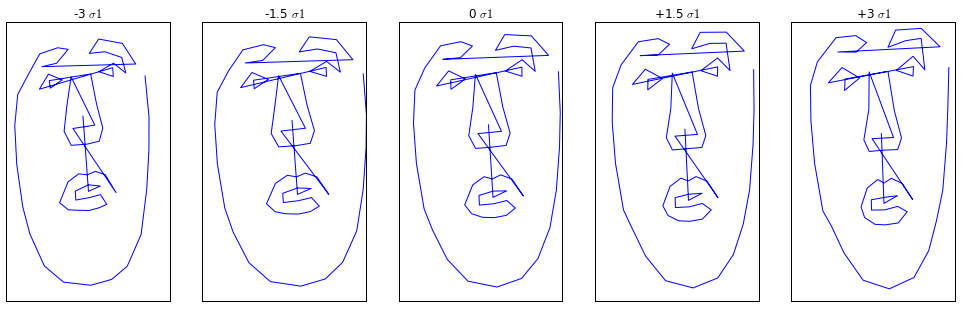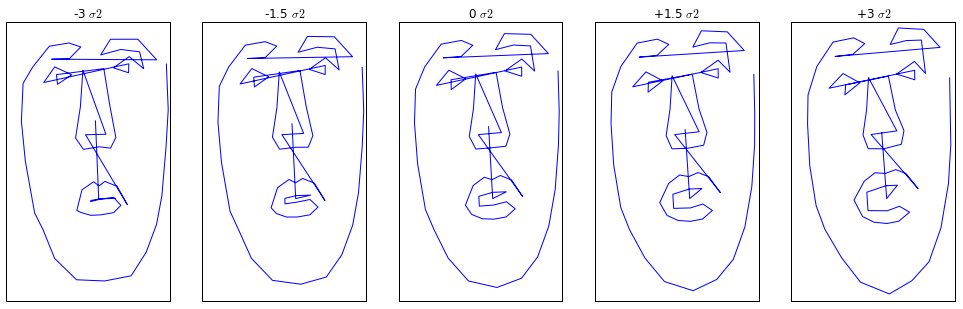### Texture Model¶

The texture is defined as the pixel intensities over the modeled entity. Here we have described the procedures to build a statistical texture model.

• Similarly to the shape model, where all the shapes are previously aligned into a common frame, the texture model requires the alignment of all texture samples to a reference texture frame also.
• The texture is mapped in a way that the control points from each sample match the control points of a suitable reference frame, the mean shape.
• Delaunay triangulation is used in the mean shape control points to establish triangles that will be used to map pixel intensities by Piece-wise Affine Warping.
• A statistical texture model is build using Principal Components Analysis, describing the texture in a condensed model.

#### Texture Mapping - Warping¶

The texture mapping is performed by using a piece-wise affine warp, i.e. partitioning the convex hull of the mean shape by a set of triangles using the Delaunay triangulation.

In mathematics and computational geometry, a Delaunay triangulation for a set P of points in a plane is a triangulation such that no point in P is inside the circumcircle of any triangle formed. Delaunay triangulation maximize the minimum angle of all the triangles in the triangulation. They tend to avoid skinny triangles.

Below we have shown the Delaunay triangulation on the set of training face images with their annotated landmarks. The concept is very simple. We will create triangles including our annotated points and then map from one triangle to another.

In :
import matplotlib.delaunay as md
plt.rcParams['figure.figsize'] = 17,8
fig=plt.figure()

for i in reversed(xrange(1,7)):
image_path="../../../data/AAM/images/%d.jpg"%i
tim_cootes=cv2.imread(image_path)
tim_cootes=cv2.cvtColor(tim_cootes, cv2.COLOR_BGR2GRAY)

dest_data=np.loadtxt('../../../data/AAM/points/%d.pts'%i, usecols=range(2))
dest_x=dest_data[:,0]
dest_y=dest_data[:,1]
_,_,dest_triangles,_=md.delaunay(dest_x, dest_y)
axes=fig.add_subplot(2,3,i)
for t in dest_triangles:
t_ext=[t, t, t, t]
axes.plot(dest_x[t_ext], dest_y[t_ext],'r')

axes.plot(dest_x,dest_y,'*')
showfig(tim_cootes, plt.get_cmap('gray'))